# DC Motor Theory

## DC Electric Circuits

• #### Question 1

If the ends of a wire loop are attached to two half-circular metal strips, arranged so that the two strips almost form a complete circle, and those strips are contacted by two “brushes” which connect to opposite poles of a battery, which way will the wire loop rotate?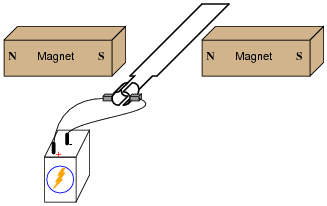• #### Question 2

When the switch closes, the ammeter will initially register a large amount of current, then the current will decay to a much lesser value over time as the motor speeds up: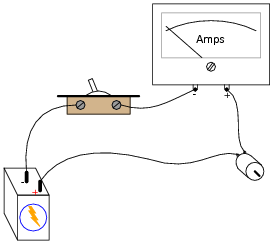In view of Ohm’s Law, where current is supposed to be a direct function of voltage and resistance (I = E/R), explain why this happens. After all, the motor’s winding resistance does not change as it spins, and the battery voltage is fairly constant. Why, then, does the current vary so greatly between initial start-up and full operating speed?

What do you think the ammeter will register after the motor has achieved full (no-load) speed, if a mechanical load is placed on the motor shaft, forcing it to slow down?

• #### Question 3

A DC electric motor spinning at 4500 RPM draws 3 amps of current with 110 volts measured at its terminals. The resistance of the armature windings, measured with an ohmmeter when the motor is at rest, unpowered, is 2.45 ohms. How much counter-EMF is the motor generating at 4500 RPM?

How much “inrush” current will there be when the motor is initially powered up (armature speed = 0 RPM), once again assuming 110 volts at the terminals?

• #### Question 4

If an electric current is passed through this wire, which direction will the wire be pushed (by the interaction of the magnetic fields)?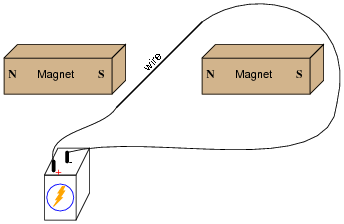Is this an example of an electric motor or an electric generator?

• #### Question 5

If we were to analyze the magnetic flux lines of a current-carrying conductor, oriented perpendicularly to a magnetic field between two bar magnets, the interaction would look something like this: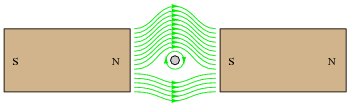This interaction of magnetic flux lines (the bar magnets’ straight lines versus the wire’s circles) will produce a mechanical force on the wire (called the Lorentz force). Which direction will this force act? Also, determine the direction of current through the conductor (seen from an end-view in the above illustration) necessary to produce the circular magnetic flux shown.

• #### Question 6

If current is passed through a loop of wire, as shown, which direction will the loop rotate?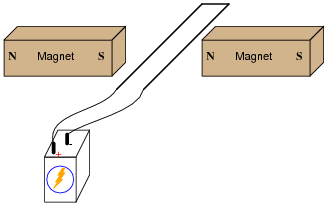• #### Question 7

A DC motor may be thought of as a series of electromagnets, radially spaced around a common shaft: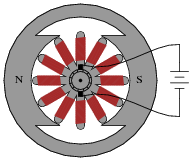This particular motor is of the “permanent magnet” type, with wire windings only on the armature.

Write the necessary magnetic polarities (“N” for north and “S” for south) on the armature’s electromagnet pole tips, in order to sustain a clockwise rotation.

• #### Question 8

Define the following DC motor terms:

• Field
• Armature
• Commutator
• Brush
• #### Question 9

When a DC motor is running, sparks may generally be seen where the carbon brushes contact the “commutator” segments. Explain why this sparking occurs, and also define the word “commutation” in its electrical usage.

What does this phenomenon indicate about the longevity of DC motors, and their suitability in certain environments?

• #### Question 10

As the armature coils in a DC motor rotate through the magnetic flux lines produced by the stationary field poles, voltage will be induced in those coils. Describe how this phenomenon relates to Faraday’s Law of electromagnetic induction, specifically in regard to what variables influence the magnitude of the induced voltage:

 e = N dφ dt

The self-induced voltage produced by a rotating armature is often called the counter-voltage, or counter-EMF. Why would it be called “counter”? What is implied by this terminology, and what electromagnetic principle is illustrated by the “counter” nature of this induced voltage?

• #### Question 11

The amount of voltage applied to a permanent-magnet DC motor, and the amount of current going through the armature windings of a permanent-magnet DC motor, are related to two mechanical quantities: maximum speed, and torque output (twisting force).

Which electrical quantity relates to which mechanical quantity? Is it voltage that relates to speed and current to torque, or visa-versa? Explain your answer.

• #### Question 12

A problem has developed in this motor circuit. When the switch is turned “on”, the motor does not turn. It does, however, draw a lot of current (several times the normal operating current) as indicated by the ammeter: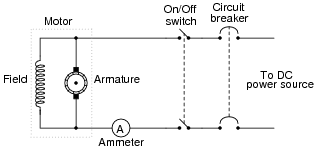Based on this information, what do you think may be wrong with the circuit? Is there anything we know for sure is not failed in the circuit? Explain your answers.